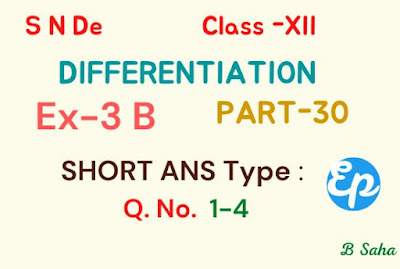# Differentiation (Part-30) | S N DeDifferentiation (Part-30) | S N De

1.  Let $~~f(x) = 4x^3+x^2-4x-1.$ The equation $~f(x) = 0~$ has roots $\,1\,$ and $\,\left(-\frac 14\right).\,$ Find the root of $~f'(x) = 0 ~$ mentioned in Rolle's  theorem.

Solution.

$~~f(x) = 4x^3+x^2-4x-1 \\ \therefore~f'(x)\\=4~\frac{d}{dx}(x^3)+\frac{d}{dx}(x^2)-4~\frac{d}{dx}(x)-\frac{d}{dx}(1)\\=4 ~\cdot 3x^2+2x-4~\cdot 1-0\\=12x^2+2x-4\\=2(6x^2+x-2)\rightarrow(1).$

Now, $~~~f'(x)=0 \\ \text{or,}~~ 2(6x^2+x-2)=0~~[\text{By (1)}] \\ \text{or,}~~ 6x^2+x-2=0 \\ \text{or,}~~ 6x^2+4x-3x-2=0 \\ \text{or,}~~ 2x(3x+2)-(3x+2)=0 \\ \text{or,}~~ (3x+2)(2x-1)=0 \\ \therefore~~ 3x+2=0 \Rightarrow x=-\frac 23 \rightarrow(2) \\ \text{and}~~2x-1=0 \Rightarrow x=\frac 12 \rightarrow(3).$

Since a polynomial function is continuous and differentiable everywhere in a finite interval, hence $\,f(x)\,$ being a polynomial is continuous in $\,[-\frac 14,1]\,$ and differentiable in $\,(-\frac 14,1)\,$ and also $~~f(-1/4)=f(1)=0.$

Now, from $~(2),(3)~$ we notice that, $~~-\frac 14 <\frac 12<1,~$ whereas $~~-\frac 23 \notin (-\frac 14,1).~$  Hence, according to Rolles's theorem, it is satisfied.

$~~\therefore~f'(x)=0~$ has a root at $~x=\frac 12.$

2.  If $~f(x)~$ is a polynomial, show that between two roots of the polynomial equation $~f(x)=0~$, there lies at least one root of the polynomial $~f'(x) = 0$.

Solution.

Let $~a~$ and $~b~$ be the two roots of the given polynomial $~f(x).~$ Then, $~~f(a)=f(b)=0.$

Since the  polynomial function  $~f(x)~$ is continuous and differentiable everywhere in a finite interval, hence  by  Rolles's theorem, we can say

$~(i)~~f(x)~$ is continuous in closed interval $~~a \leq x \leq b~~(ii)~~f(x)~~$ is differentiable in open interval $~a <x<b~~(iii)~~f(a)=f(b).$

If these three conditions are satisfied, then there exists a value $\,c\,$ in $~(a,b)~$ such that $~~f'(c)=0.$

Now, the given polynomial satisfies all these conditions of Rolle's theorem. Hence, there exists a value of $~x~$ between the roots of polynomial [i.e. between $~a~$ and $~b~$] such that $~f'(x)=0.$

### Arihant All In One Class 11th Physics, Chemistry, Biology, English Core for CBSE Exam 2024 (Set of 4 Books) Paperback

3. If $~~f(x)=(x-1)(x-2)(x-3)(x-4)~$, show that the equation $~f'(x)=0~$ has three roots $~\alpha,\beta~$ and $~\gamma~$ where $~1<\alpha <2, ~~2<\beta<3~$ and $~3 <\gamma<4$.

Solution.

$~~f(x)\\=(x-1)(x-2)(x-3)(x-4)\\=[(x-1)(x-2)]~\cdot[(x-3)(x-4)]\\=(x^2-3x+2)~\cdot (x^2-7x+12)\\=x^4-10x^3+35x^2-50x+24 \\ \therefore~ f'(x)\\=\frac{d}{dx}(x^4-10x^3+35x^2-50x+24)\\=4x^3-30x^2+70x-50.$

Now, $~~f'(x)=0 \\ \text{or,}~~ 4x^3-30x^2+70x-50=0 \\ \text{or,}~~ 2(2x^3-15x^2+35x-25)=0 \\ \text{or,}~~ 2x^3-15x^2+35x-25=0 \\ \text{or,}~~ 2x^3-5x^2-10x^2+25x\\~~~~~+10x-25=0 \\ \text{or,}~~ x^2(2x-5)-5x(2x-5)\\~~~~~+5(2x-5)=0 \\ \text{or,}~~ (2x-5)(x^2-5x+5)=0 \\ \therefore 2x-5=0 \Rightarrow x=\frac 52=2.5 \rightarrow(1) \\ \text{and}~~x^2-5x+5=0 \\ \text{or,}~~x=\frac{-(-5) \pm \sqrt{(-5)^2-4 \cdot 5 \cdot 1}}{2 \cdot 1} \\ \text{or,}~~x=\frac{5 \pm \sqrt{25-20}}{2} \\ \text{or,}~~x= \frac{5 \pm \sqrt 5}{2} \\ \text{or,}~~x= \frac{5 + \sqrt 5}{2},~\frac{5 - \sqrt 5}{2}\\ \text{or,}~~x= 3.62,~1.38 \rightarrow(2)$

Hence, from $~(1)~$ and $~(2)~$ we notice that

$~~1<1.38<2,~2<2.5<3,~3<3.62<4. \\ \therefore \alpha=1.38,~\beta=2.5,~\gamma=3.62.$

So, finally we can conclude that the equation $~f'(x)=0~$ has three roots $~\alpha,\beta~$ and $~\gamma~$ where $~1<\alpha <2, ~~2<\beta<3~$ and $~3 <\gamma<4$.

$~4.~~$ Show that the equation $~e^{x-1}+x-2=0~$ has no real root

which is less than $\,1$.

Solution.

Clearly, $~x=1~$ satisfies the given equation $~e^{x-1}+x-2=0~$.

We assume that $~f(x)=e^{x-1}+x-2=0~$, has a real root $\,\alpha\,$ other than $~1~.$ We may suppose that $~~\alpha>1.~$ The case $~~(\alpha<1~)~$ is exactly similar.

Now, applying the Rolle's theorem on $~[\alpha,1]~$, we get $~\beta \in(1,\alpha)~$ such that $~~f'(\beta)=0.$

In case, $~\alpha<1,~$ we apply the theorem on $~[\alpha,1].$

Now, $~f'(x)=e^{x-1}+1 \\ \therefore f'(\beta)= 0 \\ \Rightarrow e^{\beta-1}=-1 \rightarrow(1)$

Hence, from $\,(1)\,$ we get a result which is not possible. So, we can conclude that the equation $~e^{x-1}+x-2=0~$ has no real root

which is less than $\,1$.